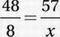# SAT Math Multiple Choice Question 723: Answer and Explanation

### Test Information

Question: 723

3. A 48-gram serving of breakfast cereal contains 8 grams of sugar. How many grams of sugar are there in a 57-gram serving of the same cereal?

• A. 9.5
• B. 10.5
• C. 11.5
• D. 12.5

Explanation:

A

Problem Solving/Data Analysis (proportions) EASY

Set up a proportion:Cross multiply:

456 = 48x

Divide by 48:

9.5 = x